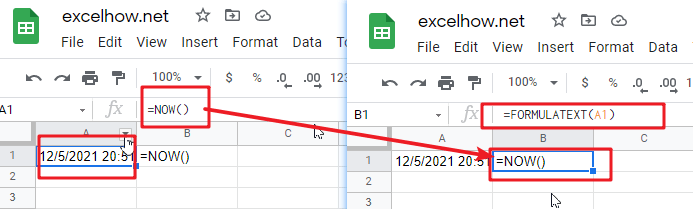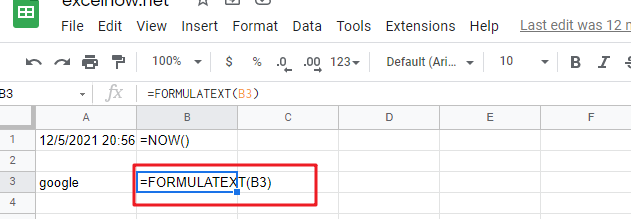# ExcelHow

This post will guide you how to use Google Sheets FORMULATEXT function with syntax and examples.

## Description

The Google Sheets FORMULATEXT function returns a formula as string. You can get a formula as a text string from a given cell reference.

The FORMULATEXT function can be used to extract the formula as text from a cell in google sheets. The purpose of this function is to get the formula in a cell and the returned value is the formula as text.

The FORMULATEXT function is a build-in function in Google Sheets and it is categorized as a Lookup function.

## Syntax

The syntax of the FORMULATEXT function is as below:

=FORMULATEXT (cell)

Where the FORMULATEXT function arguments are:

• cell -This is a required argument. The reference to cell or a cell range.

Note:

• FORMULATEXT function only take one arugument, it can be a cell reference.
• If Cell doesn’t contain a formula, the FORMULATEXT function returns #N/A error.
• FORMULATEXT function returns what is displayed in the formula bar when selecting a cell.
• If a cell range is passed into FORMULATEXT function, only the top left most cell is extracted.

## Google Sheets FORMULATEXT Function Examples

The below examples will show you how to use google sheets FORMULATEXT Function to extract a formula as a string.

#1 if the cell A1 contain one formula =NOW(), and you wish to extract this formula in cell A1 as a text string, just using the FORMULATEXT function:

`=FORMULATEXT(A1)`#2 the cell A3 contain one string “google”, and using the FORMULATEXT function to extract a formula in Cell C3, it will returns #N/A error, type:

`=FORMULATEXT(C1)`#3 If the cell passed into FORMULATEXT references the cell that contains the FORMULATEXT formula, it can handle this kind of circular reference, type:

`=FORMULATEXT(B3)`